# Which is the correct equilibrium constant expression for the following reaction? 2 NaHCO3(s) Na2CO3(s) + CO2(g)...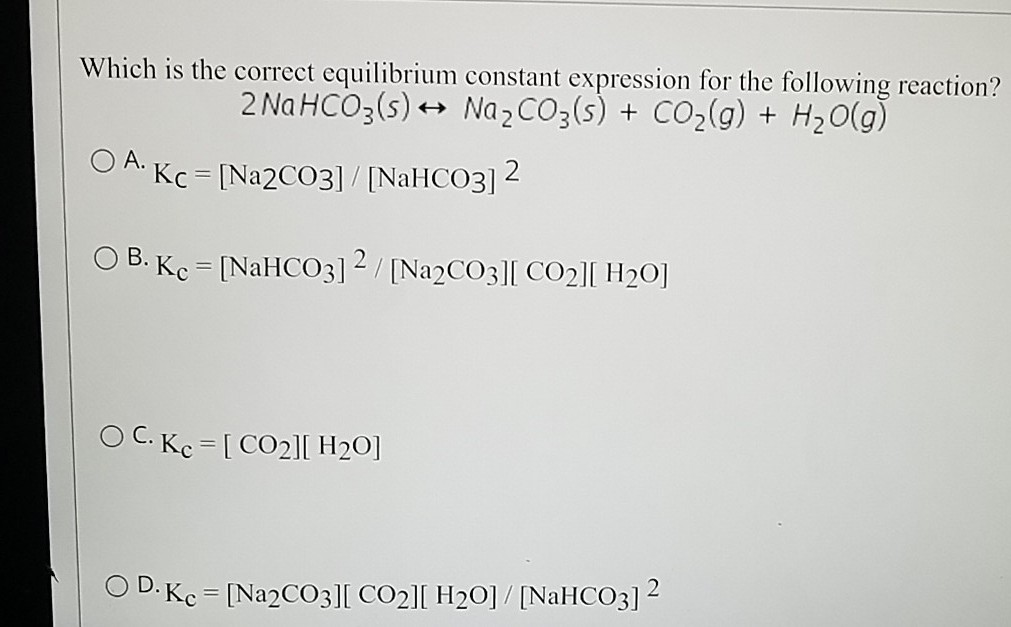Which is the correct equilibrium constant expression for the following reaction? 2 NaHCO3(s) Na2CO3(s) + CO2(g) + H2O(g) O A. Kc=[Na2CO3] / [NaHCO3)2 O B. Kc = [NaHCO3] 2 / [Na2CO3][ CO2][ H20] O C. Kc = [ CO2][ H20] OD. Kc = [Na2CO3][ CO2][ H20] / [NaHCO3)2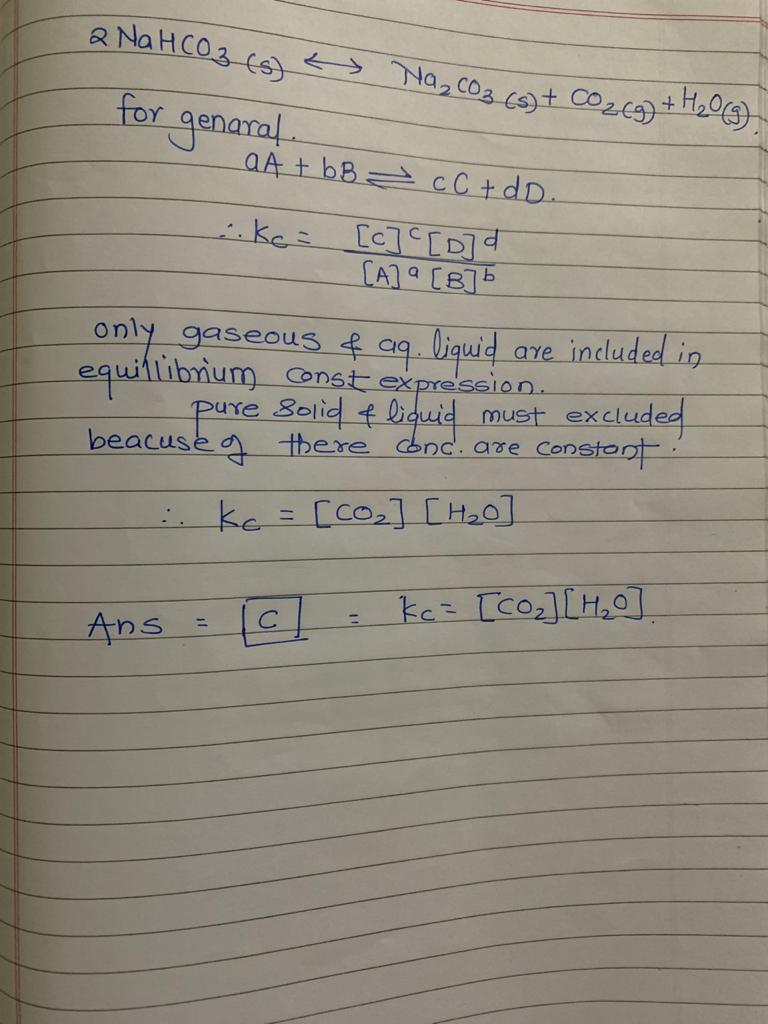#### Earn Coin

Coins can be redeemed for fabulous gifts.

Similar Homework Help Questions
• ### Which is the correct equilibrium constant expression for the following reaction? 2 NaHCO3(s) +- Na2CO3(s) +...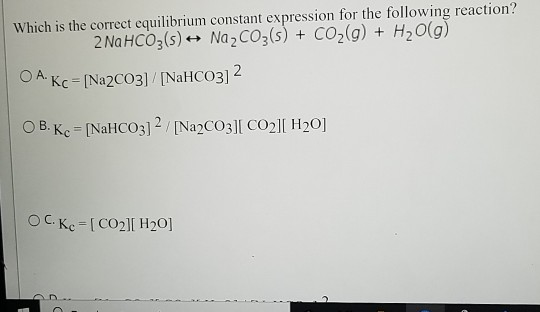Which is the correct equilibrium constant expression for the following reaction? 2 NaHCO3(s) +- Na2CO3(s) + CO2(g) + H2O(g) O A. Kc = [Na2CO3]/[NaHCO3)2 O B. Kc = [NaHCO3]2/[Na2CO3][ CO2][ H20] OC. Kc = [CO2][ H20]

• ### At 125 degrees C, the equilibrium constant Kp for the following reaction is 0.25. 2 NaHCO3...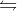At 125 degrees C, the equilibrium constant Kp for the following reaction is 0.25. 2 NaHCO3 (s) Na2CO3(s) + CO2(g) + H2O(g) a.) Calculate Kc for the reaction at 125 degrees C b.) At the beginning of the reaction given in part (A) only 10.0g of NaHCO3 was there in an evacuated 1.00L flask. What is the total pressure in the flask, after the equilibrium is established 125 degrees C?

• ### Consider the following reaction at equilibrium: NiO(s)+CO(g) 2 Ni(s) + CO2(g). Select all of the following...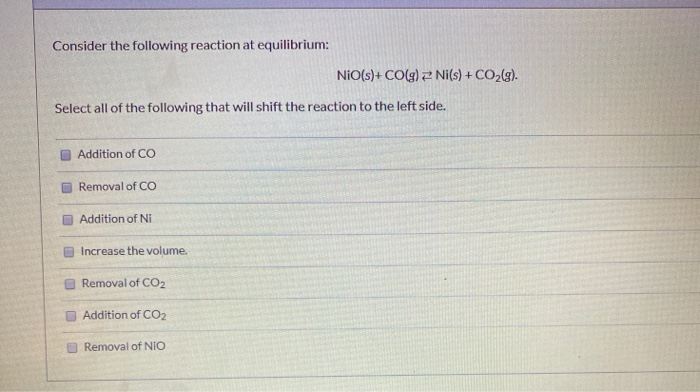Consider the following reaction at equilibrium: NiO(s)+CO(g) 2 Ni(s) + CO2(g). Select all of the following that will shift the reaction to the left side. Addition of CO Removal of CO Addition of Ni Increase the volume. Removal of CO2 Addition of CO2 Removal of NIO Question 1 What is the expression for equilibrium constant for the following reaction? 2 H2S(g) + SO2(g) = 35(s) + 2 H2O(g) Kc= [HQ][S] [SO][H251 Kc= [HO] [H,872 Kc=  [H2 51 (H20 Kc=...

• ### What would be the equilibrium constant expression for the following reaction? Na2CO3 (s) + 2 HCl...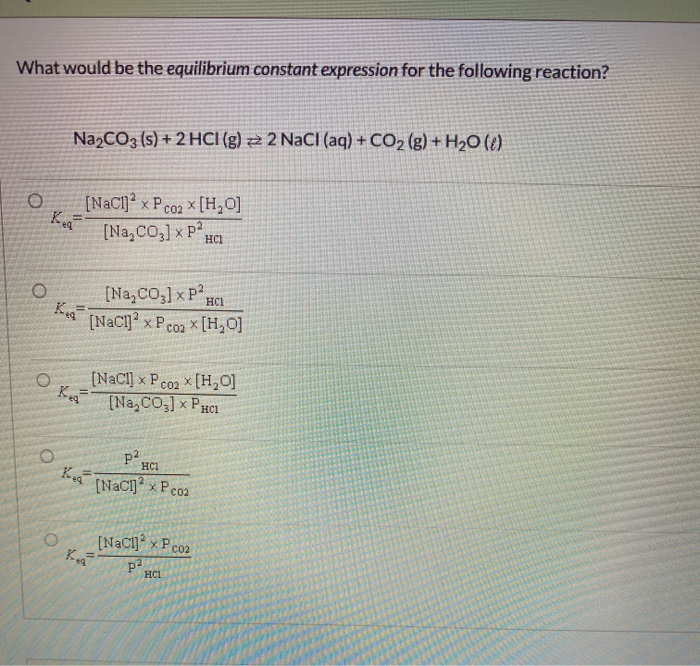What would be the equilibrium constant expression for the following reaction? Na2CO3 (s) + 2 HCl (g) z 2 NaCl(aq) + CO2(g) + H20 (8) o Keg [NaCl] x P co2 x [H0] [Na, C03] x Pa HCI O HCI [Na, C03] x P2 [NaCl]" x P co2 * [H, 0] O CO2 Reg [NaCl] x PC X[H0] [Na, C03] ⓇPH HCl p2 HCl [NaCl]- XP Coz [NaCl] XP Co2 P2 HCl

• ### Possible Chemical Equations NaHCO3 (s) → NaOH (s) CO2(g) NaHCO3 (s) → Na2CO3(s) + CO2(g) +...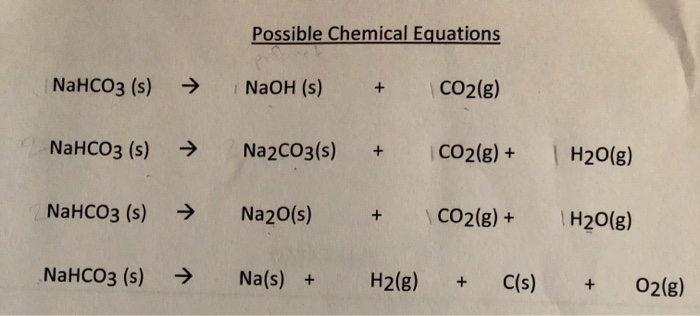Possible Chemical Equations NaHCO3 (s) → NaOH (s) CO2(g) NaHCO3 (s) → Na2CO3(s) + CO2(g) + H2O(g) NaHCO3 (s) → Na2O(s) + CO2(g) + H2O(g) NaHCO3 (s) → Na(s) + H2(g) + C(s) + O2(g) Conclusions: 1. Rewrite and balance each of the four chemical equations from the lab introduction.

• ### Possible Chemical Equations NaHCO3 (s) → NaOH (s) CO2(g) NaHCO3 (s) → Na2CO3(s) + CO2(g) +...Possible Chemical Equations NaHCO3 (s) → NaOH (s) CO2(g) NaHCO3 (s) → Na2CO3(s) + CO2(g) + H2O(g) NaHCO3 (s) → Na2O(s) + CO2(g) + H2O(g) NaHCO3 (s) → Na(s) + H2(g) + C(s) + O2(g) Conclusions: 1. Rewrite and balance each of the four chemical equations from the lab introduction.

• ### Which of the following processes have a ΔS > 0? A) Na2CO3(s) + H2O(g) + CO2(g) → 2 NaHCO3(s) B)CH4(g) + H2O(g) → CO(g...

Which of the following processes have a ΔS > 0? A) Na2CO3(s) + H2O(g) + CO2(g) → 2 NaHCO3(s) B)CH4(g) + H2O(g) → CO(g) + 3 H2(g) C)CH3OH(l) → CH3OH(s) D)N2(g) + 3 H2(g) → 2 NH3(g) E)All of the above processes have a DS > 0.

• ### 1.Sodium hydrogen carbonate, NaHCO3, can be decomposed completely by heating. 2 NaHCO3(s) → Na2CO3(s) + CO2(g)...

1.Sodium hydrogen carbonate, NaHCO3, can be decomposed completely by heating. 2 NaHCO3(s) → Na2CO3(s) + CO2(g) + H2O(g) A sample of impure NaHCO3 with an initial mass of 0.739 g yielded a solid residue (consisting of Na2CO3 and other solids) with a final mass of 0.564 g. Determine the mass percent of NaHCO3 in the sample. 2.In a process for producing acetic acid, oxygen gas is bubbled into acetaldehyde, CH3CHO, containing manganese(II) acetate (catalyst) under pressure at 60°C. 2CH3CHO(l) +...

• ### Consider the following reaction and its equilibrium constant: 4 CuO(s) + CH4(g)  ⇌  CO2(g) + 4 Cu(s) +...

Consider the following reaction and its equilibrium constant: 4 CuO(s) + CH4(g)  ⇌  CO2(g) + 4 Cu(s) + 2 H2O(g)         Kc = 1.10 A reaction mixture contains 0.38 M CH4, 0.96 M CO2 and 0.14 M H2O.  Which of the following statements is TRUE concerning this system? The system is at equilibrium. The reaction will shift in the direction of reactants. The reaction quotient will increase. The equilibrium constant will increase. The reaction will shift in the direction of products.

• ### Which of the following expressions is the correct equilibrium-constant expression for the reaction below? 3 Fe(s)...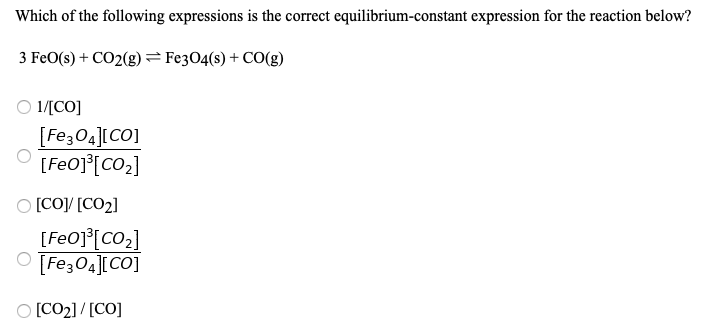Which of the following expressions is the correct equilibrium-constant expression for the reaction below? 3 Fe(s) + CO2(g) = Fe3O4(s) + CO(g) 1/[CO] [Fe304][CO] [FO]*[CO2] O [Coy [CO2] [Feo][CO2] [Fe3O4][CO] O [CO2]/[CO]

Free Homework App